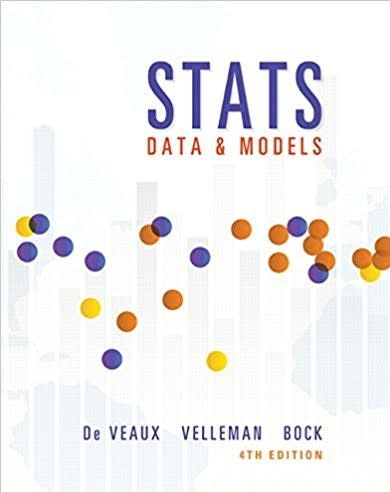Statistics Stats: Data and Models# Stats: Data and Models DeVeaux • 4th Edition •

Not the textbook you were looking for? Pick another one here.

Qualitative Data

## Ch.3 - Displaying and Summarizing Quantitative Data

Quantitative Data Measures of Central Tendency Measures of Spread: Standard Deviation Measures of Spread: Interquartile Range Boxplots (The Five Number Summary)

## Ch.5 - The Standard Deviation as a Rule and the Normal Model

The Normal Distribution The Z-Score The Z-Table Probabilities from Scores Scores from Probability

## Ch.13 - From Randomness to Probability

Probability Notations

## Ch.14 - Probability Rules!

Addition Rule Multiplication Rule Advanced Applications of Probability Rules

## Ch.15 - Random Variables

Discrete Random Variable Discrete Random Variable Mean and Standard Deviation

## Ch.16 - Probability Models

The Binomial Distribution The Binomial Formula The Binomial Table

## Ch.17 - Sampling Distribution Models

Sampling Distribution for a Sample Proportion Sampling Distribution for a Sample Mean

## Ch.18 - Confidence Intervals for Proportions

Confidence Interval for a Population Proportion

## Ch.19 - Testing Hypotheses about Proportions

The Idea Behind Hypothesis Testing Null and Alternative Hypotheses Critical Values & Rejection Regiona Test Statistic: Proportion

## Ch.20 - Inferences about Means

Large Sample Confidence Interval for a Population Mean Small Sample Confidence Interval for a Population Mean Sample Size Large Sample Test Statistic: Mean Small Sample Test Statistic: Mean

## Ch.21 - More About Tests and Intervals

Type 1 & Type 2 Errors